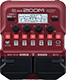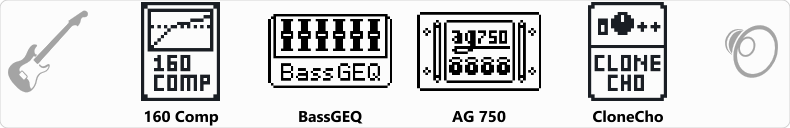# basic bass 1

Discussion in 'Zoom B1/B1X Four' started by alejandro rodas martinez, Aug 1, 2022.

1. ### alejandro rodas martinezNew Member

basic bass 1Device: Zoom B1 Four
Firmware: 2.00

Name on device: H.basico
Optimized for: Phones/Speaker

Effects chain:basico para tocar todo genero. generic bass sound .

Effect: "160 Comp" (Dynamics), active - "yes"
"Threshold" = -14
"Ratio" = 3.3
"Knee" = SOFT
"Volume" = 61

Effect: "BassGEQ" (Filter), active - "yes"
"50" = 5.5
"120" = 6.5
"400" = 3.5
"500" = 5.0
"800" = 5.0
"4.5k" = 4.5
"10k" = 4.0
"VOL" = 76

Effect: "AG 750" (Amp simulator), active - "yes"
"Bass" = 54
"Middle" = 81
"Treble" = 73
"Gain" = 57
"Bright" = On
"Deep" = On
"Volume" = 64

Effect: "CloneCho" (Modulation), active - "yes"
"Depth" = 2
"Rate" = 15
"Tone" = 85
"Mix" = 59

Patch Volume: 61